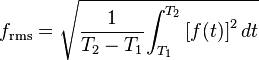# Sinusoidal Waveforms

## Homework Statement

Hi,

The question is " Are the following statements Sinusoidal? And are they Periodic?
I have produced the waveforms in MathCad
The limits are 0 - 4Pi

1.f(x)= sinx + 2sin(x-(Pi/6))
2.f(x)= sinx +sin2x
3.f(x)= sinx + 1/2(sin2x)
4.f(x)= sinx + 1/3(sin3x)+1/5(sin5x)
5.f(x)= sinx.cos2x
6.f(x)= sin3x.sin2x
7.f(x)= sin^23x

## The Attempt at a Solution

I think they are all periodic as my understanding of periodic is that the waveform repeats itself after 2pi (360 degrees) Which they all seem to do.
I am unsure about the Sinusoidal part though. I know that number 1 is but not sure about the rest!! How do I tell?

I also have to work out the r.m.s value of a complex waveform, Am I right in saying that if its sinusoidal I can use Vmax/root2? If its not sinusoidal can I use this formula?Last edited:

Astronuc
Staff Emeritus
Periodic means that the function repeats the same value 'periodically', i.e. with the same period, i.e. f(t) = f(t+T) = f(t+nT) where n is an integer, and T is the period.

Integating the sin function over one period gives 0.

See - http://en.wikipedia.org/wiki/Sine_wave

A sinusoidal function certainly is one that can be put in the general form

$$y(t) = sin (\omega t + \theta)$$, where $\omega$ is the angular frequency (rad/sec) = 2$\pi$ f, and $\theta$ is the phase or phase shift in radians.

Hi,

Thanks for the reply. I get the periodic part now (Many Thanks)

However I am still somewhat confused about the Sinusoidal part. All the formulas mention Time or frequency, however I only seem to have a phase figure. (i.e) I have 0 - 4Pi plotted along the horizontal axis and Amplitude along the vertical axis in my graphs.

Am I missing something blindingly obvious?

Many Thanks